# Texas Go Math Grade 1 Lesson 18.3 Answer Key Time to the Hour and Half Hour

Refer to our Texas Go Math Grade 1 Answer Key Pdf to score good marks in the exams. Test yourself by practicing the problems from Texas Go Math Grade 1 Lesson 18.3 Answer Key Time to the Hour and Half Hour.

## Texas Go Math Grade 1 Lesson 18.3 Answer Key Time to the Hour and Half Hour

Explore
Each clock has an hour hand and a minute hand. Use what you know about the hour hand to write the unknown numbers.It is 1:00.
The hour hand points to the _________.
The minute hand points to the _________.
It is half past 1:00 or 1:30.

Explanation:The hour hand points between the 1 and the 2.
The minute hand points to the 6.

FOR THE TEACHER • Have children look at the two docks and tell how much time has passed.
Time is 1:30 or half past 1.

Explanation:
The hour hand points between the 1 and the 2.
The minute hand points to the 6.

Math Talk
Mathematical Processes
Look at the top clock. Explain how you know which is the minute hand.
I know its minute hand just by its length. Long hand is called as minute hand in the clock.

Explanation:
Long hand is called as minute hand in the clock.
Short hand is called as hour hand in the clock.

Model and Draw
An hour has 60 minutesThe clock shows 10:00.

Explanation:
Hour hand is showing 10.
Minute hand is showing 12.
The clock is showing the time: 10:00.

A half hour has 30 minutes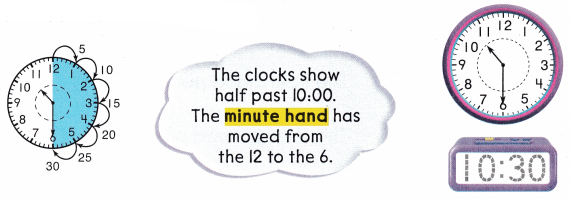The clock is showing the time: 10:30 or half past 10.

Explanation:
Hour hand is showing 10.
Minute hand is showing 6.
The clock is showing the time: 10:30 or half past 10.

Share and Show
Write the time.
Question 1.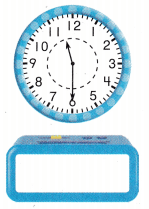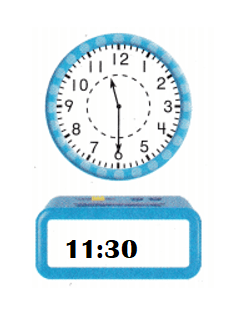Explanation:
Hour hand is showing 11.
Minute hand is showing 6.
The clock is showing the time: 11:30 or half past 11.

Question 2.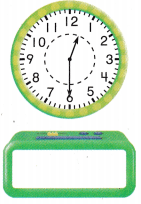Explanation:
Hour hand is showing 12.
Minute hand is showing 6.
The clock is showing the time: 12:30 or half past 12.

Question 3.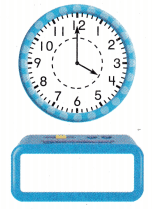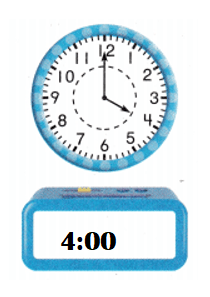Explanation:
Hour hand is showing 4.
Minute hand is showing 12.
The clock is showing the time: 4:00.

Problem Solving
Write the time.
Question 4.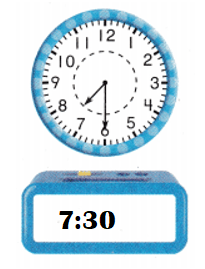Explanation:
Hour hand is showing 7.
Minute hand is showing 6.
The clock is showing the time: 7:30 or half past 7.

Question 5.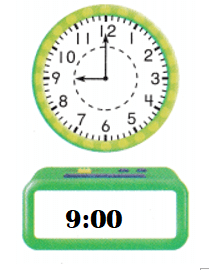Explanation:
Hour hand is showing 9.
Minute hand is showing 12.
The clock is showing the time: 9:00.

Question 6.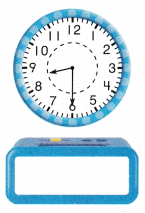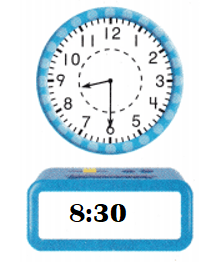Explanation:
Hour hand is showing 8.
Minute hand is showing 6.
The clock is showing the time: 8:30 or half past 8.

Question 7.
Sara goes to the park when both the hour hand and the minute hand point to the 12. What time does Sara go to the park?
1 o’clock
12 o’clock
2 o’clock
Time Sara goes to the park = 12:00.
12 o’clock

Explanation:
Sara goes to the park when both the hour hand and the minute hand point to the 12.
=> Time Sara goes to the park = 12:00.

Question 8.
Mel goes to the park at half past 3. Which time shows when Mel goes to the park?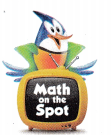3:00
6:00
3:30
Time at Mel goes to the park = 3:30 or half past 3.
3:30.

Explanation:
Mel goes to the park at half past 3.
=> Time at Mel goes to the park = 3:30 or half past 3.

Question 9.
H.O.T. Multi-Step The hour hand points halfway between the 2 and 3. Draw the hour hand and the minute hand. Write the time.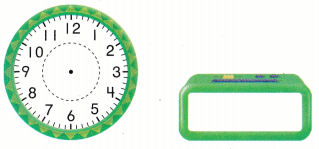Explanation:
The hour hand points halfway between the 2 and 3.
=> 2:30 or half past 2.

Question 10.
The clock shows the time that Ken gets home from her soccer game. What time does the clock show?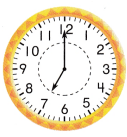(A) 7:00
(B) 7:30
(C) 8:00
Time shown in the clock = 7:00.
(A) 7:00.

Explanation:
Time shown in the clock = 7:00.Question 11.
Which clock shows 11:30?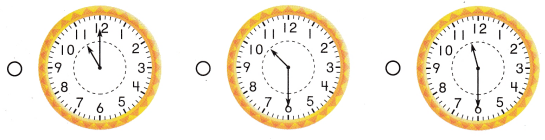Explanation:
First clock shows the time: 11:00
Second clock shows the time: 10:30 or half past 10.
Third clock shows the time: 11:30 or half past 11.

Question 12.
Sean goes to the library when the hour hand points to the 6, and the minute hand points to the 12. What time does Sean go to the library?
(A) 6:00
(B) 6:30
(C) 7:30
Time at Sean goes to the library = 6:00.
(A) 6:00.

Explanation:
Sean goes to the library when the hour hand points to the 6, and the minute hand points to the 12.
=> Time at Sean goes to the library = 6:00.

Question 13.
Texas Test Prep What time is it?(A) 6:30
(B) 7:30
(C) 8:00
Time shown in the clock = 7:30 or half past 7.
(B) 7:30

Explanation:
Time shown in the clock = 7:30 or half past 7.

TAKE HOME ACTIVITY • At times on the half hour, have your child show you the minute hand and the hour hand on a clock, and tell what time it is.
My kid shown the hours hand showing 7 and minutes hand showing 6.
Time shown in the clock = 7:30 or half past 7.

Explanation:My kid shown the hours hand showing 7 and minutes hand showing 6.

### Texas Go Math Grade 1 Lesson 18.3 Homework and Practice Answer Key

Write the time.
Question 1.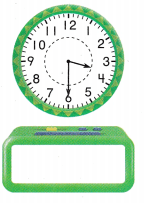Explanation:
Time shown in the clock = 3:30 or half past 3.

Question 2.Explanation:
Time shown in the clock = 6:00.

Question 3.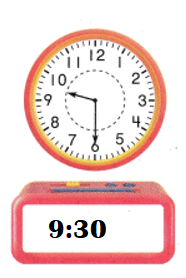Explanation:
Time shown in the clock = 9:30 or half past 9.

Problem Solving
Question 4.
Luis goes bowling at 11:00. Which time shows when Luis goes bowling?
11 o’clock
12 o’clock
2 o’clockExplanation:
Luis goes bowling at 11:00.
=> Time Luis goes bowling = 11:00.

Question 5.
Multi-Step The hour hand points halfway between 8 and 9. Draw the hour hand and the minute hand. Write the time.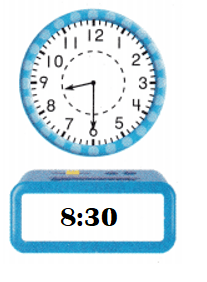Explanation:
The hour hand points halfway between 8 and 9.
=> Time the clock shows = 8:30 or half past 8.

Lesson Check
Question 6.
The clock shows the time that Ken gets home from his karate lesson. What time does the clock show?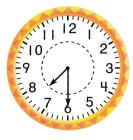(A) 7:30
(B) 8:30
(C) 8:00
Time shown in the clock = 7:30 or half past 7.
(A) 7:30.

Explanation:
The clock shows the time that Ken gets home from his karate lesson.
Time shown in the clock = 7:30 or half past 7.Question 7.
Which clock shows 10:30?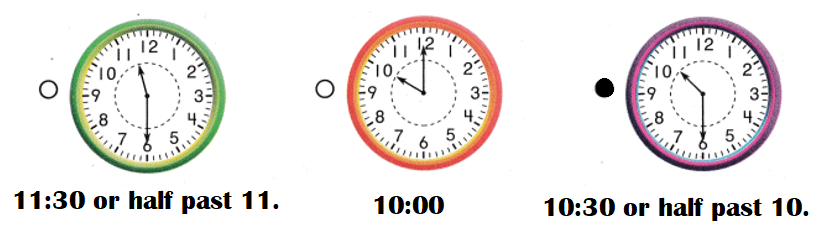Explanation:
First clock shows the time: 11:30 or half past 11.
Second clock shows the time: 10:00.
Third clock shows the time: 10:30 or half past 10.

Question 8.
What time is it?(A) 5:00
(B) 4:30
(C) 5:30
Time shown in the clock = 4:30 or half past 4.
(B) 4:30.

Explanation:
Time shown in the clock = 4:30 or half past 4.Question 9.
Sara goes to the park when the hour hand points to the II, and the minute hand points to the 12. What time does Sara go to the park?
(A) 11:00
(B) 12:00
(C) 11:30